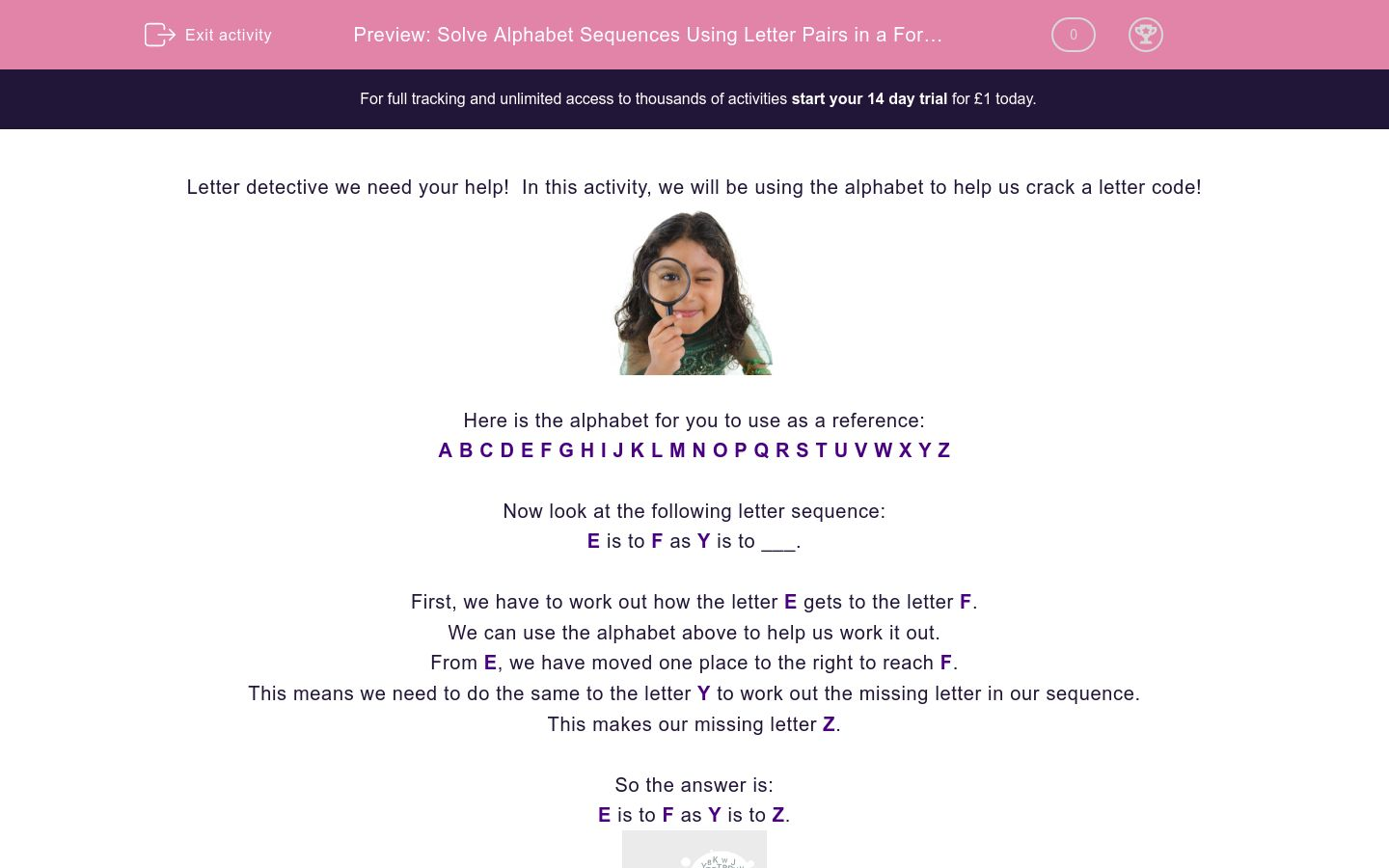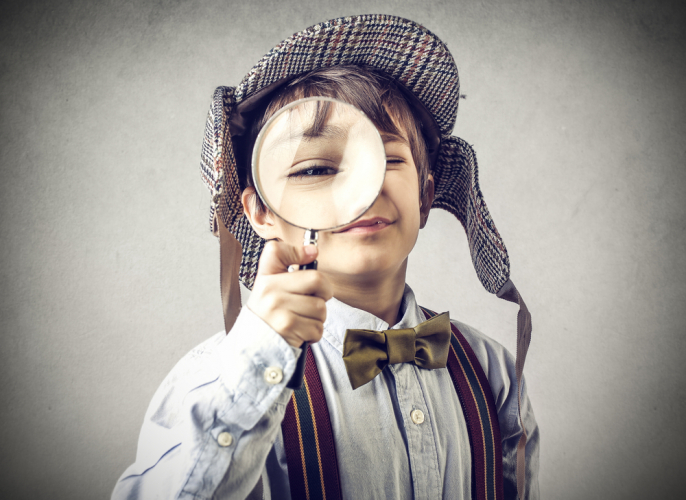# Solve Alphabet Sequences Using Letter Pairs in a Forward Direction Only

In this worksheet, students will solve alphabetical sequences involving single letter pairs in a forward motion only. It will develop students’ problem solving and sequencing skills.Key stage:  KS 2

Curriculum topic:   Verbal Reasoning

Curriculum subtopic:   Letter Connections

Difficulty level:### QUESTION 1 of 10

Letter detective we need your help!  In this activity, we will be using the alphabet to help us crack a letter code!Here is the alphabet for you to use as a reference:

A B C D E F G H I J K L M N O P Q R S T U V W X Y Z

Now look at the following letter sequence:

E is to F as Y is to ___.

First, we have to work out how the letter E gets to the letter F.

We can use the alphabet above to help us work it out.

From E, we have moved one place to the right to reach F.

This means we need to do the same to the letter Y to work out the missing letter in our sequence.

This makes our missing letter Z.

E is to F as Y is to Z.Let’s try another:

A is to C as F is to ___.

First, we have to work out how we get from the letter A to the letter C.

If we look at the alphabet above, we can see that we need to move 2 places to the right.

So we need to do the same with the letter F.

And the missing letter is H.

A is to C as F is to H.

In this activity, you will need to be a sequence superstar and complete the letter pairs.

Remember to use the alphabet line to help you work out the code.

Here are two letter sequences that both follow the same pattern:

H is to M as R is to ______ ?A  B  C  D  E  F  G  H  I  J  K  L  M  N  O  P  Q  R  S  T  U  V  W  X  Y  Z

Here are two letter sequences that both follow the same pattern:

C is to G as K is to ______ ?

 A  B  C  D  E  F  G  H  I  J  K  L  M  N  O  P  Q  R  S  T  U  V  W  X  Y  Z

Here are two letter sequences that both follow the same pattern:G is to O as F is to ______ ?

 A  B  C  D  E  F  G  H  I  J  K  L  M  N  O  P  Q  R  S  T  U  V  W  X  Y  Z

Here are two letter sequences that both follow the same pattern:

L is to S as N is to ______ ?

 A  B  C  D  E  F  G  H  I  J  K  L  M  N  O  P  Q  R  S  T  U  V  W  X  Y  Z

Here are two letter sequences that both follow the same pattern:

G is to P as B is to ______ ?

 A  B  C  D  E  F  G  H  I  J  K  L  M  N  O  P  Q  R  S  T  U  V  W  X  Y  Z

Here are two letter sequences that both follow the same pattern:

G is to G as L is to ______ ?A  B  C  D  E  F  G  H  I  J  K  L  M  N  O  P  Q  R  S  T  U  V  W  X  Y  Z

Here are two letter sequences that both follow the same pattern:

J as U as is E to ______ ?

 A  B  C  D  E  F  G  H  I  J  K  L  M  N  O  P  Q  R  S  T  U  V  W  X  Y  Z

Here are two letter sequences that both follow the same pattern:

C is to Q as E is to ______ ?A  B  C  D  E  F  G  H  I  J  K  L  M  N  O  P  Q  R  S  T  U  V  W  X  Y  Z

Here are two letter sequences that both follow the same pattern:

C is to R as F is to ______ ?A  B  C  D  E  F  G  H  I  J  K  L  M  N  O  P  Q  R  S  T  U  V  W  X  Y  Z

Here are two letter sequences that both follow the same pattern:

H is to N as F is to ______ ?

 A  B  C  D  E  F  G  H  I  J  K  L  M  N  O  P  Q  R  S  T  U  V  W  X  Y  Z
• Question 1

Here are two letter sequences that both follow the same pattern:

H is to M as R is to ______ ?A  B  C  D  E  F  G  H  I  J  K  L  M  N  O  P  Q  R  S  T  U  V  W  X  Y  Z
W
EDDIE SAYS
How did you find the first question, detective? Each sequence follows the order of the alphabet going forwards, with five jumps each time. So five jumps after R is W.
• Question 2

Here are two letter sequences that both follow the same pattern:

C is to G as K is to ______ ?

 A  B  C  D  E  F  G  H  I  J  K  L  M  N  O  P  Q  R  S  T  U  V  W  X  Y  Z
O
EDDIE SAYS
Each sequence follows the order of the alphabet going forwards, with four jumps each time. So, four jumps after K is O. Great!
• Question 3

Here are two letter sequences that both follow the same pattern:G is to O as F is to ______ ?

 A  B  C  D  E  F  G  H  I  J  K  L  M  N  O  P  Q  R  S  T  U  V  W  X  Y  Z
N
EDDIE SAYS
Fantastic effort! This time both sequences follow the order of the alphabet going forwards, with eight jumps each time. Eight jumps after F is N.
• Question 4

Here are two letter sequences that both follow the same pattern:

L is to S as N is to ______ ?

 A  B  C  D  E  F  G  H  I  J  K  L  M  N  O  P  Q  R  S  T  U  V  W  X  Y  Z
U
EDDIE SAYS
Both sequences follow the order of the alphabet going forwards, with seven jumps each time. Seven jumps after N is U. Good work, detective!
• Question 5

Here are two letter sequences that both follow the same pattern:

G is to P as B is to ______ ?

 A  B  C  D  E  F  G  H  I  J  K  L  M  N  O  P  Q  R  S  T  U  V  W  X  Y  Z
K
EDDIE SAYS
You're halfway through this activity! Both sequences follow the order of the alphabet going forwards, with nine jumps each time. Nine jumps after B is K.
• Question 6

Here are two letter sequences that both follow the same pattern:

G is to G as L is to ______ ?A  B  C  D  E  F  G  H  I  J  K  L  M  N  O  P  Q  R  S  T  U  V  W  X  Y  Z
L
EDDIE SAYS
Double trouble! This time there have been no jumps, the cheeky letters are the same each time! Did we catch you out, detective?!
• Question 7

Here are two letter sequences that both follow the same pattern:

J as U as is E to ______ ?

 A  B  C  D  E  F  G  H  I  J  K  L  M  N  O  P  Q  R  S  T  U  V  W  X  Y  Z
P
EDDIE SAYS
A lot of jumps this time! Both sequences follow the order of the alphabet going forwards, with eleven jumps each time. If you take eleven jumps from E, you reach P.
• Question 8

Here are two letter sequences that both follow the same pattern:

C is to Q as E is to ______ ?A  B  C  D  E  F  G  H  I  J  K  L  M  N  O  P  Q  R  S  T  U  V  W  X  Y  Z
S
EDDIE SAYS
Did you keep up with the jumps?! Both sequences follow the order of the alphabet going forwards, with fourteen jumps each time. Fourteen jumps after E is S.
• Question 9

Here are two letter sequences that both follow the same pattern:

C is to R as F is to ______ ?A  B  C  D  E  F  G  H  I  J  K  L  M  N  O  P  Q  R  S  T  U  V  W  X  Y  Z
U
EDDIE SAYS
Both sequences follow the order of the alphabet going forwards, with fifteen jumps each time. Fifteen jumps after F is U.
Phew, with that many jumps I think those letters need a lie down! See you on the next activity, detective!
• Question 10

Here are two letter sequences that both follow the same pattern:

H is to N as F is to ______ ?

 A  B  C  D  E  F  G  H  I  J  K  L  M  N  O  P  Q  R  S  T  U  V  W  X  Y  Z
L
EDDIE SAYS
This sequence follows the order of the alphabet going forwards, with six jumps each time. Six jumps after F is L.
Time to have a go at spotting the final sequence!
---- OR ----

Sign up for a £1 trial so you can track and measure your child's progress on this activity.

### What is EdPlace?

We're your National Curriculum aligned online education content provider helping each child succeed in English, maths and science from year 1 to GCSE. With an EdPlace account you’ll be able to track and measure progress, helping each child achieve their best. We build confidence and attainment by personalising each child’s learning at a level that suits them.

Get started